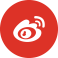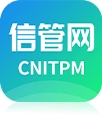﻿ 信息系统项目管理师案例分析每日一练试题（2021/12/29） - 每日一练 - 信管网

# 信管网每日一练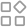导航

## 信息系统项目管理师案例分析每日一练试题（2021/12/29）

2021年12月30日来源：信管网 作者:cnitpm【问题1】(10分)

【问题2】(3分)

【问题3】 (2分)

【问题4】 (6分)

A.销售费用

B.项目成员的工资

C.办公室电费

D.项目成员的差旅费

E.项目所需的物料费

F.公司为员工缴纳的商业保险费

bac=30000＜br＞ac=19700＜br＞pv=25500＜br＞ev=20000＜br＞cv=ev-ac=300＜br＞sv=ev-pv=-5000＜br＞cpi=ev/ac=1.02＜br＞spi=ev/pv=0.78＜br＞etc=bac-ev/cpi=30000-20000/1.02=9803.9

ev=1000+1500+3500+800+2300+4500＊0.8+2200+2500＊0.6+4200＊0.5+3000＊0.5=20000 ac=19700 cv=ev-ac=300 sv=ev-pv=20000-25500=-5500 成本控制良好，低。 进度滞后。 cpi=ev/ac=20000/19700=1.0 spi=ev/pv=20000/25500=0.7 etc= bac不变 直接成本：bde 间接成本：acf

1.ev=20000，pv=25500，ac=19700；cv=ev-ac=300,sv=ev-pv=-5500；cpi=1.01；spi=0.78；项目成本节约，进度落后 2.etc=bac/spi=38461,5 3. 4.直接成本 ade 间接费用 bcf

（1）: pv=25500, ev=20000, ac=19700, cv=ev-ac=300, sv=ev-pv=-5500, cpi=ev/ac=1.02 spi=ev/pv=0.78 项目成本结余，进度落后。 （2）: 典型，etc=（bac-ev）/cpi=9019.6元 （3） 没有变化 （4） : 直接成本：b,d,e 简介成本：a,c,f

•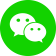•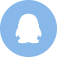•